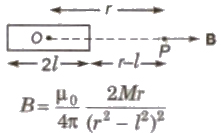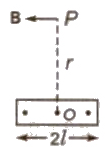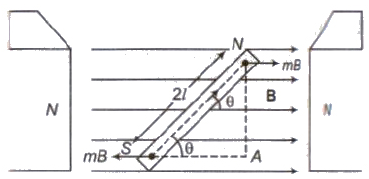Courses

# Magnetic Dipole Class 12 Notes | EduRev

## Class 12 : Magnetic Dipole Class 12 Notes | EduRev

The document Magnetic Dipole Class 12 Notes | EduRev is a part of the Class 12 Course Physics Class 12.
All you need of Class 12 at this link: Class 12

Magnetic Dipole

Magnetic dipole is an arrangement of two unlike magnetic poles of equal pole strength separated by a very small distance, e.g., a small bar magnet, a magnetic needle, a current carrying loop etc.

Magnetic Dipole Moment

The product of the distance (2 l) between the two poles and the pole strength of either pole is called magnetic dipole moment.

Magnetic dipole moment

M = m (2 l)

Its SI unit is ‘joule/tesla’ or ‘ampere-metre2‘.

Its direction is from south pole towards north pole.

Magnetic Field Due to a Magnetic Dipole

(1) On Axial LineIf r > > l, then

B = μo / 4 π 2M / r3

(ii) On Equatorial LineB = μo / 4 π M / (r2 + l2)3 / 2

If r > > l, then

B = μo / 4 π 2M / r3

Torque Acting on a Magnetic Dipole

When a Magnetic Dipole (M) is placed in a uniform magnetic field (B), then a Torque acts on it, Which is given byτ = M * B

or τ = MB sin θ

Where θ is angle between the dipole axis and magnetic field.

Potential Energy of a Magnetic Dipole in a Uniform Magnetic Field

The work done in rotating the dipole against the action of the torque is stored as potential energy of the dipole.

Potential Energy, U = W = – MB cos θ = – M . B

Offer running on EduRev: Apply code STAYHOME200 to get INR 200 off on our premium plan EduRev Infinity!

,

,

,

,

,

,

,

,

,

,

,

,

,

,

,

,

,

,

,

,

,

;